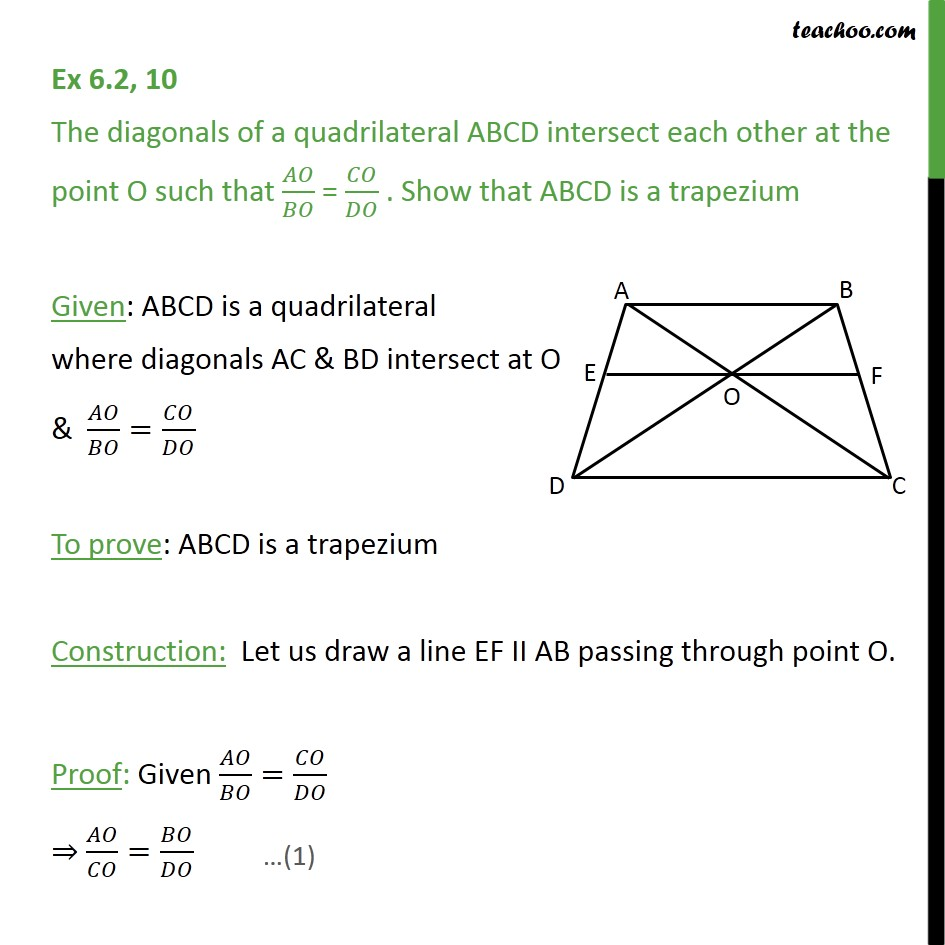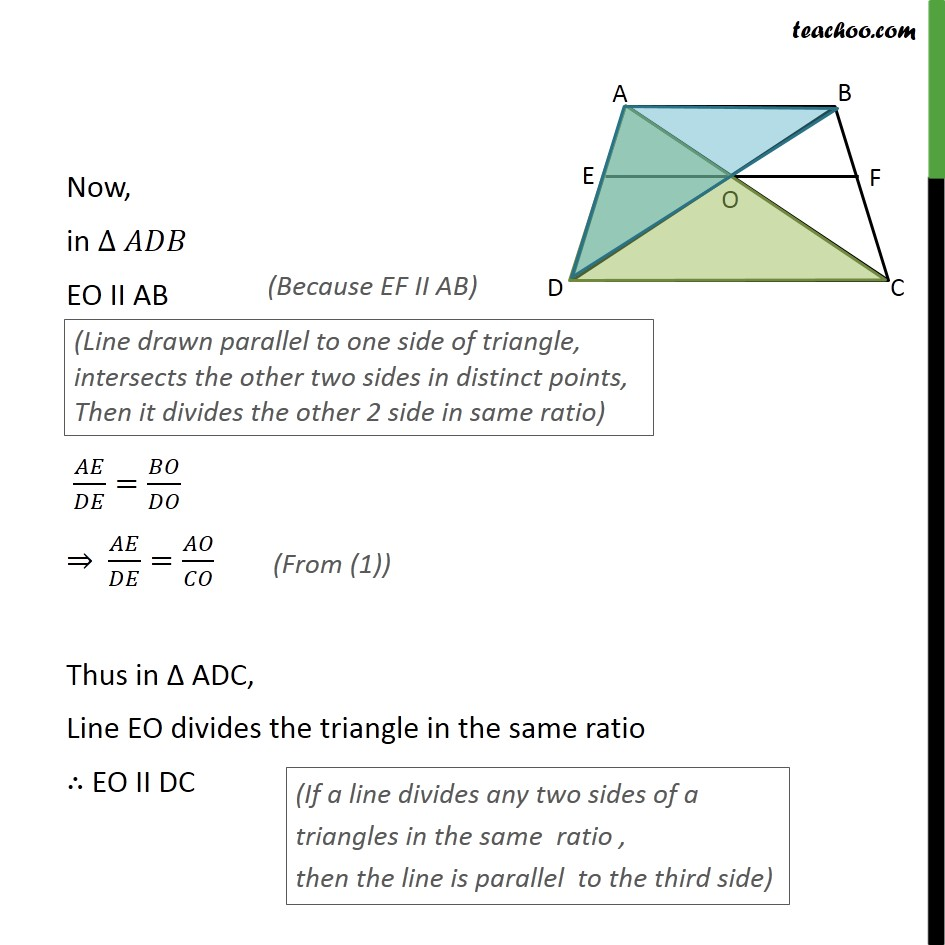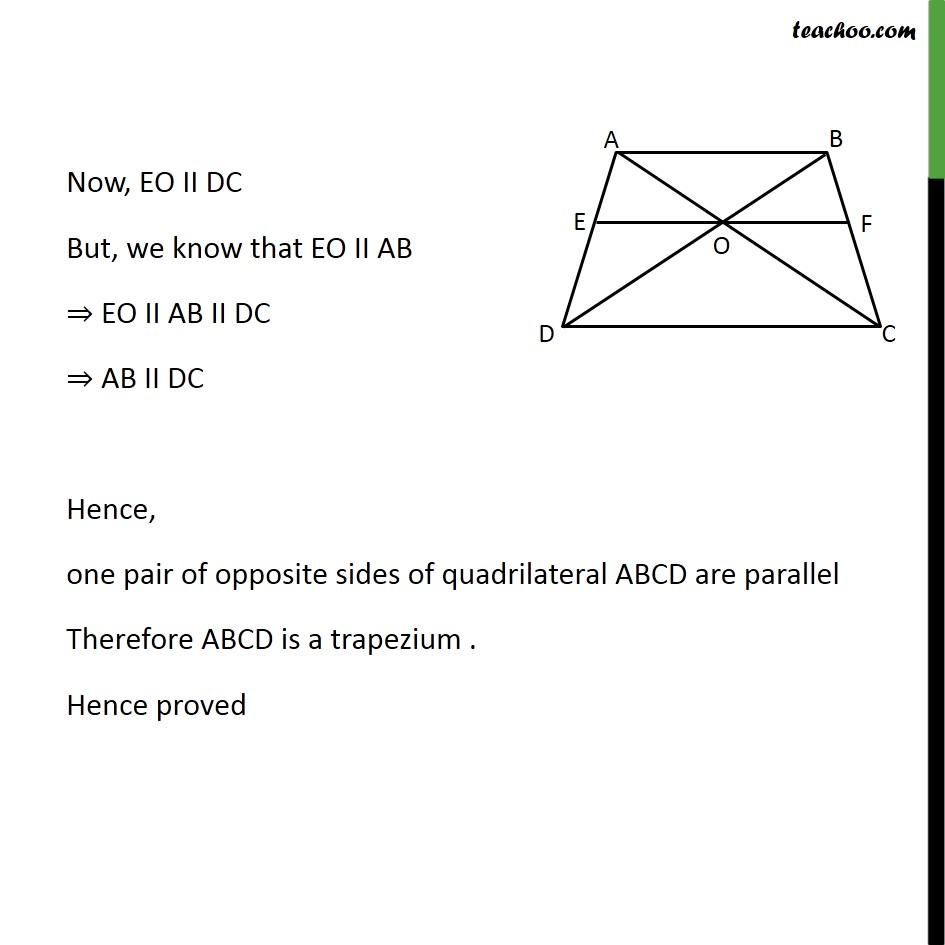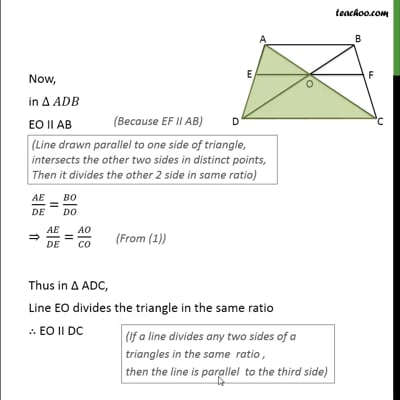Ex 6.2

Chapter 6 Class 10 Triangles
Serial order wiseThis video is only available for Teachoo black users

Learn in your speed, with individual attention - Teachoo Maths 1-on-1 Class

### Transcript

Ex 6.2, 10 The diagonals of a quadrilateral ABCD intersect each other at the point O such that 𝐴𝑂/𝐵𝑂 = 𝐶𝑂/𝐷𝑂 . Show that ABCD is a trapezium Given: ABCD is a quadrilateral where diagonals AC & BD intersect at O & 𝐴𝑂/𝐵𝑂=𝐶𝑂/𝐷𝑂 To prove: ABCD is a trapezium Construction: Let us draw a line EF II AB passing through point O. Proof: Given 𝐴𝑂/𝐵𝑂=𝐶𝑂/𝐷𝑂 ⇒ 𝐴𝑂/𝐶𝑂=𝐵𝑂/𝐷𝑂 Now, in ∆ 𝐴𝐷𝐵 EO II AB 𝐴𝐸/𝐷𝐸=𝐵𝑂/𝐷𝑂 ⇒ 𝐴𝐸/𝐷𝐸=𝐴𝑂/𝐶𝑂 Thus in Δ ADC, Line EO divides the triangle in the same ratio ∴ EO II DC Now, EO II DC But, we know that EO II AB ⇒ EO II AB II DC ⇒ AB II DC Hence, one pair of opposite sides of quadrilateral ABCD are parallel Therefore ABCD is a trapezium . Hence proved